\$B:#>,\$N0lGU(B

\$BKhF|!":#>,\$N0lGU\$r>e\$2\$F\$\$\$^\$9!#(B
\$B:#>,0{\$`\$b\$N\$K:\$\$C\$?\$i!";29M\$K\$7\$F\$_\$F\$/\$@\$5\$\$!#(B

2019\$BG/(B8\$B7n(B

 1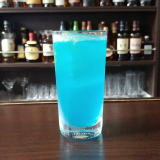(I;O0%=62(B 2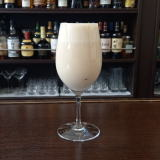(IM^2X0=^%PY8(B 3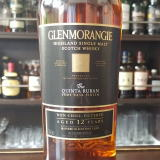(I8^Z]S0Z]<^(B 4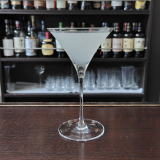(IO21P%K^0A(B 5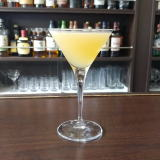(IO21P%K^0A(B 6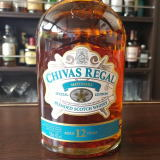(I<0J^=%X06^Y%P=^EW(B 7(I;^;^(B 8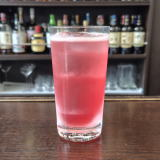(IZ/D^Q0](B 9(I 10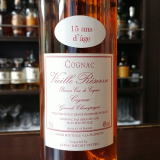(IN_0Y%<^[0(B15\$BG/(B 11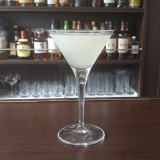(ID)Y0%[O]=(B 12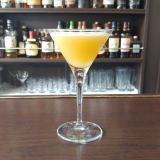(I<^,/8%2]%;^%N^/8=(B 13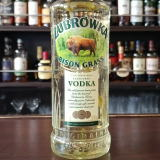(I=^L^[/6(B 14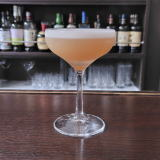(I8[0J^0%8WL^(B 15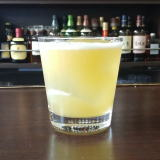(I1Y:^]7](B 16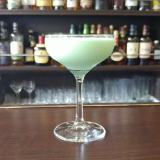(I8^X0]%K_0=(B 17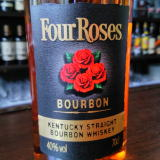(IL+1%[0>^=^%L^W/8(B 18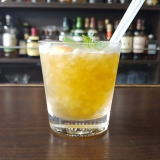(IL^W]C^0%=O/<-(B 19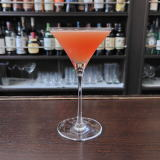(I1Y6N_H(B 20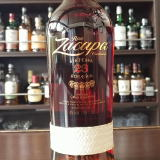(I[]%;6J_(B 21(I1]<^0(B 22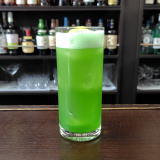(I8^X0]%C(0%80W0(B 23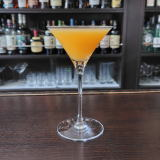(I3^*HA1]%;]>/D(B 24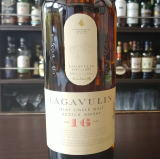(IW6^3^0X](B16\$BG/(B 25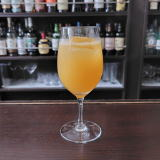(IJ\21]%J_]A(B 26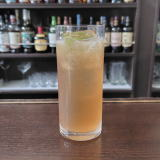(IJ^/8%<^.0]=^(B 27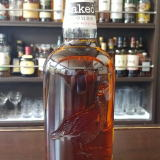(IH27/D^%8^W3=(B 28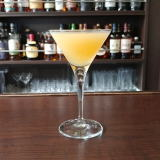(IL^[/8(B&(IL+0Y(B 29(IL[0=^]%@^27X(B 30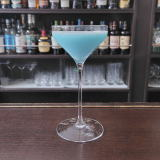(IN\2DE2D(B 31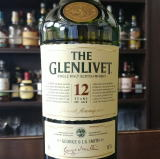(I8^Z]XM^/D(B12\$BG/(B